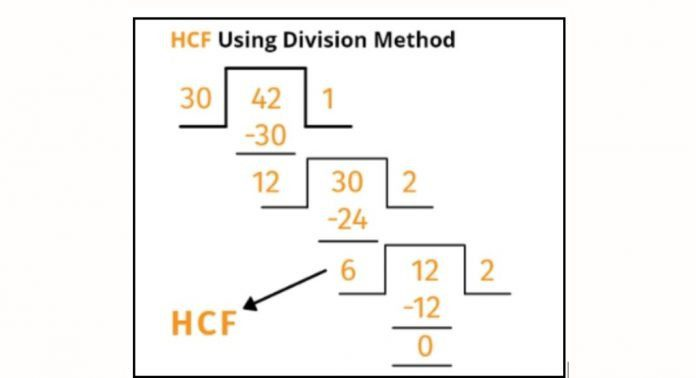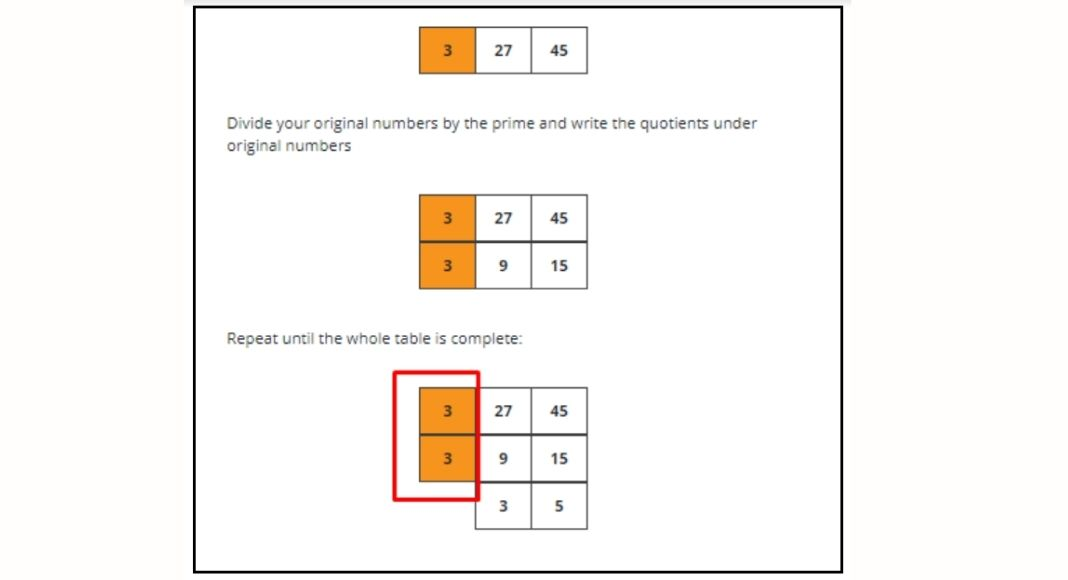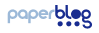# Why do We Need to Learn to Find The Highest Common Factor?The highest common factor and least common factor are amongst the studies which usually start from the grade forth and continue till the education of Universities end. This is because it has importance at different stages of life especially when a complex calculation comes.

Most of the students go for learning the HCF and LCM without understanding the reason to learn it. However, in this article, we will tell you some of the uses of the highest common factor in daily life that can make your complex problems solved.

## What is HCF and Why we need to find it?

The largest common number between the factors of two or more numbers is known as the highest common factor while it is also known as the greatest common factor. It has different important points in our life which becomes the reason to learn it properly.

For example, if you have some large number and you want to make in the ratio format then the highest common factor could be used to divide them to find the least ratio possibility. Moreover, when we have to give a sequence then the highest common factor could be used to make the sequence better. For example, if you give the sitting arrangement to the examination hall and there are different subjects then the HCF factor can help you in arrangements.

Read More: How to Write A Philosophy Essay?

Most of the architects and analysts use this term in their work to design the projects better. For example, if they want to make the map of thousands of kilometers on a paper which is in meters then they would use the highest common factor value to divide the kilometers and convert it into the centimeters.

### How do we calculate the highest common factor?

To calculate the highest common factor of two or more numbers, two common ways are usually used including the manual calculation as well as the online calculators. In manual, the calculation might become complex because the factorization of the large number might take a lot of steps but in online calculators, the solution becomes quite easy.

The greatest common factor calculator usually takes seconds over a single click to generate the highest common factor by different methods.

Here are some of the manual ways to calculate the highest common factor:

• Division Method

One of the easiest ways to find the HCF of multiple numbers is to use the division method. The division method is considered as easy because the factor is finding through step by step factorization.

In this method, you have to divide the number which is larger as compared to the other with the smallest one. In the next step, the divisor would be the new dividend. Repeat the process until the remainder is zero. However, once the remainder is finalized, the last divider will be considered as HCF as shown in the picture below:

• Factor Tree

This method is also known as the prime factorization method because, in this step, you have to find the prime factors of the number for which you are finding the greatest common factor. In the first step of this method, you have to make the factors of each number including the occurrences of all the numbers. Now, multiple occurrences and the result would be the highest common factor.

Here is an example of finding the HCF of 27 and 45 using factor tree or prime factorization:

The prime factors of 27 are: 3 x 3 x 3

The prime factors of 45 are: 3 x 3 x 5

Now, the common occurrences of 27 and 45 are: 3, 3

The highest common factor is: 3 x 3 = 9

• Upside Down Division

This is another to find the highest common factor which is also an easy way to solve your factorization. In this method, you have to write the numbers side by side. Now, think of a number that is a common factor as well as the prime number. Remember, the number should divide all the numbers without making any remainder.

Now, divide the numbers by this prime number and write quotients. Repeat the process until the remainders are not divisible by the same number. Now, multiple all the dividers and the result would be the highest common factor. Here is an example of how to make the greatest common factor using the Upside down division method.

To find the HCF of 27 and 45, the number would be 3 which is prime and common to both the numbers.Now, multiplying these dividers would give you the highest common factor as 9.

• Factorization method

The most used way to find the highest common factor is to use the list of factors. This is the easiest way because you just have to make the factors of each number. After that, you have to note the common numbers between these factors and note which number is the largest. The largest number would be the highest common factor in these numbers.

Here is the example of the factorization method for finding the HCF:

The factorization of 32 are 1, 2, 4, 8, 16, 32

The factorization of 48 are 1, 2, 4, 6, 8, 12, 16, 24, 48

The common factors are 1, 2, 4, 8, 16

The highest common factor is 16